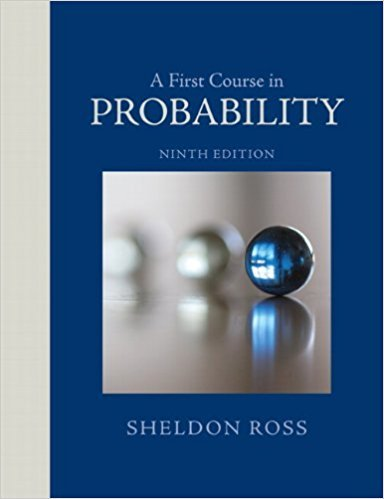×
Log in to StudySoup
Get Full Access to A First Course In Probability - 9 Edition - Chapter 4 - Problem 2ste
Join StudySoup for FREE
Get Full Access to A First Course In Probability - 9 Edition - Chapter 4 - Problem 2ste

Already have an account? Login here
×
Reset your password

# Suppose that X takes on one of the values 0, 1, and 2. IfISBN: 9780321794772 63

## Solution for problem 2STE Chapter 4

A First Course in Probability | 9th Edition

• Textbook Solutions
• 2901 Step-by-step solutions solved by professors and subject experts
• Get 24/7 help from StudySoup virtual teaching assistantsA First Course in Probability | 9th Edition

4 5 1 362 Reviews
11
1
Problem 2STE

Problem 2STE

Suppose that X takes on one of the values 0, 1, and 2. If for some constant c,P{X = i} = cP{X = i− 1}, i = 1, 2, find E[X].

Step-by-Step Solution:

Answer

Step 1 of 1

(a)

Suppose thattakes on one of the valuesIf for some constantWe are asked to findWe have givenThis relationship implies thatwhereBecause these probabilities sum to 1, we can write,We know the expectation of a random variableHenceisStep 2 of 1

##### ISBN: 9780321794772

Unlock Textbook Solution

Enter your email below to unlock your verified solution to:

Suppose that X takes on one of the values 0, 1, and 2. If